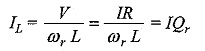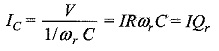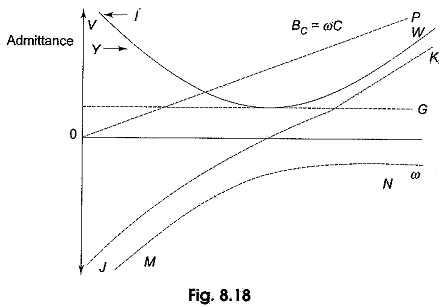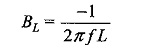## Current Magnification in Parallel Resonance:

Current Magnification in Parallel Resonance occurs when the voltage applied to the parallel circuit, V=IR

SinceFor the Capacitor,Therefore, the quality factor Qr = IL/I or IC/I

### Reactance curves in Parallel Resonance:

The effect of variation of frequency on the reactance of the parallel circuit is shown in Fig. 8.18.The effect of inductive susceptance,Inductive susceptance is inversely proportional to the frequency or ω. Hence it is represented by a rectangular hyperbola, MN. It is drawn in fourth quadrant, since BL is negative. Capacitive susceptance, BC = 2πfC. It is directly proportional to the frequency f or ω. Hence it is represented by OP, passing through the origin. Net susceptance B = BC – BL. It is represented by the curve JK, which is a hyperbola. At point ωr, the total susceptance is zero, and resonance takes place.

The variation of the admittance Y and the current I is represented by curve VW. The current will be minimum at resonant frequency.

Scroll to Top